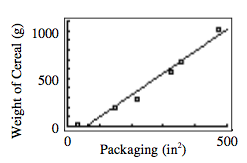### Home > CCA > Chapter 7 > Lesson 7.1.4 > Problem7-57

7-57.

In problem 6-10, Battle Creek Cereal was considering a variety of packaging options for Toasted Oats cereal. They wish to predict the net weight of cereal based on the amount of cardboard used for the package. Below is a list of six current packages.

Packaging cardboard (in2)

Net weight of cereal (g)

$34$

$21$

$150$

$198$

$218$

$283$

$325$

$567$

$357$

$680$

$471$

$1020$

checksum $1555$

checksum $2769$

1. In a previous lesson, you may have hand-drawn a line of best fit for this data. Now use your calculator to find the equation of the LSRL. Sketch the scatterplot.$y = 0.118x^{\left(1.467\right)}$